# SSAT Middle Level Math : How to add fractions

## Example Questions

### Example Question #121 : Fractions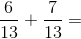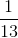Explanation:

Add the numerators and keep the denominator; then, reduce: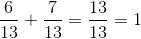Answer:### Example Question #41 : How To Add Fractions

Solve: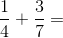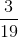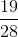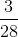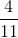Explanation:

The least common denominator ofandis.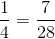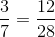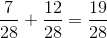### Example Question #42 : How To Add Fractions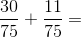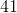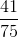Explanation:

Add the numerators and keep the denominator the same: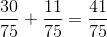Answer:### Example Question #43 : How To Add Fractions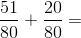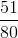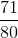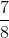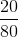Explanation:

Add the numerators and keep the denominator the same: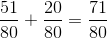Answer:### Example Question #44 : How To Add Fractions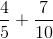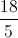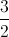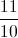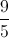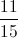Explanation:

First, we need to find a common denominator. In this case, we can convert both denominators to.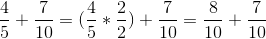Now we can add the numerators.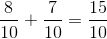Both the numerator and denominator are divisible by, so we can simplify.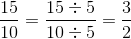### Example Question #45 : How To Add Fractions

Add: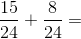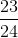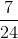Explanation:

To solve, add the numerators and leave the denominators the same: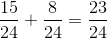Answer:### Example Question #46 : How To Add Fractions

Add: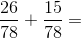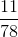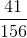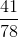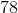Explanation:

To solve, add the numerators and leave the denominators the same: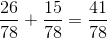Answer:### Example Question #47 : How To Add Fractions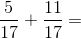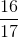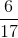Explanation:

Add the numerators and keep the denominator the same: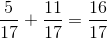Answer:### Example Question #221 : Fractions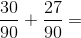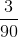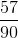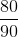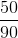Explanation:

Add the numerators and keep the denominator the same: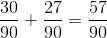Answer:### Example Question #49 : How To Add Fractions

Solve the equation.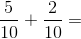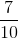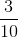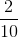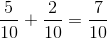Answer: